## How to impute missing values?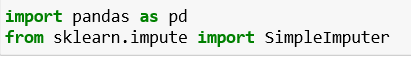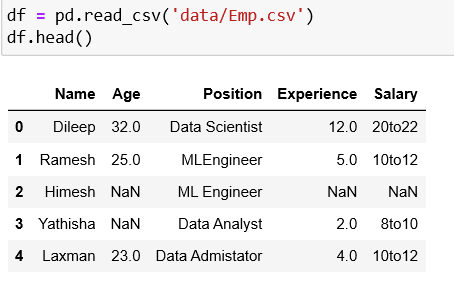We can check missing values in a dataframe using two built-in functions, `pandas.DataFrame.isna()` and `pandas.DataFrame.isnull()` as follows: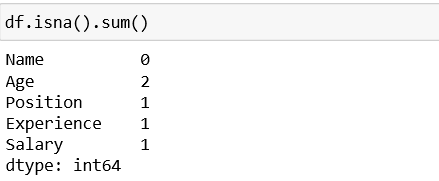The features Age, Position, Experience and Salary are having missing value.

The missing data can be handled in two ways:

Dropping a row with missing data.

Replacing NaN with data.

1. Dropping a Row With Missing Data

As stated above, missing data row can be ignored but it can lead to inconsistent results because the data that was removed can be important for further data analysis. Even though, it is not a recommended approach, but we can go with it when the dataset is quite large. The rows with the missing data values may have a very small impact in large dataset.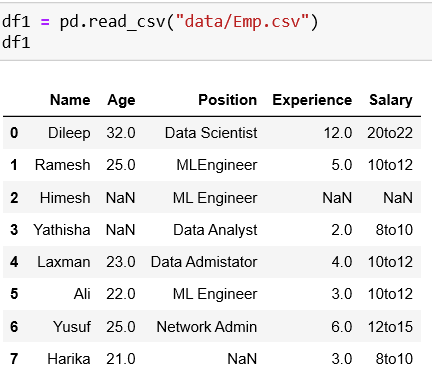We can consider following scenarios to drop rows with NaNs:

drop all rows that have any NaN values

drop only if entire row has NaN values

drop only if a row has more than 2 NaN values

drop NaN in a specific column

Case 1: Drop all rows that have NaN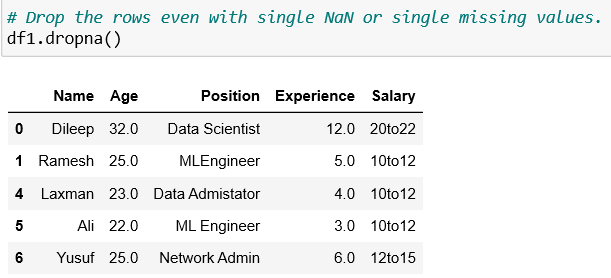The above code removes all the rows of a column that has NaN values.

Case 2: Drop only if entire row has NaN values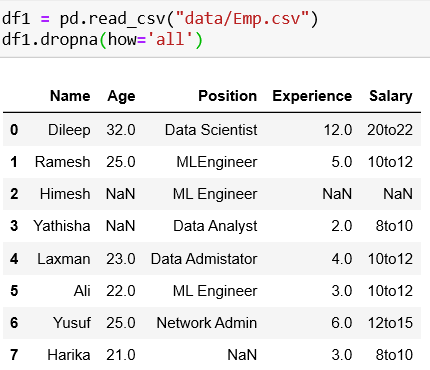Case 3: Drop only if a row has more than 2 NaN values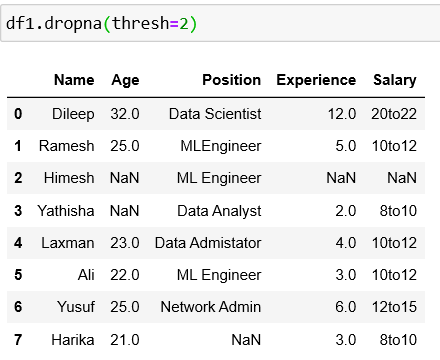Case 4: Drop NaN in a specific column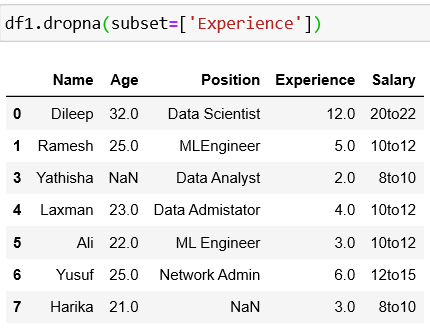We should not use this method, despite its easiness. Let’s see alternative methods to make the dataset more consistent.

Replacing NaN With Data

Imputation is an alternative approach to resolve to handle missing data. The missing data values are substituted by another statistically computed value. There could be scenarios where the dataset is small or each row of the dataset represents a important value. So, we cannot remove the row from the dataset in such cases. The missing values can be imputed.

There are several methods to define the substitute for the missing value. The value can be filled by the following values:

The `mean value` of the other column values.

The `median value` of the other column values.

Fill with the `mode value` (most frequent) in the dataset.

Fill with the `constant value` in the dataset.

We can use Scikit’s Imputer class to impute missing data values . The constructor of this class takes the following arguments as input:

`missing_values`: This is the actual value that needs to be filled. The programmer can specify the value that needs to be considered for substitute.

`strategy`: We can use strategies such as mean, median, constant and most_frequent and replace the missing data value.

`axis`: This argument takes either 0 or 1 as input value. 0 represent a row and 1 represents a column.

`verbose`: It defines the verbosity of the imputer. The default value is 0.

`Copy`: By default, it is set to true, which signifies that a copy of the original object is created. Otherwise, not.

Let’s look at how to fill the missing values with the different strategies with examples.

1. Imputing with mean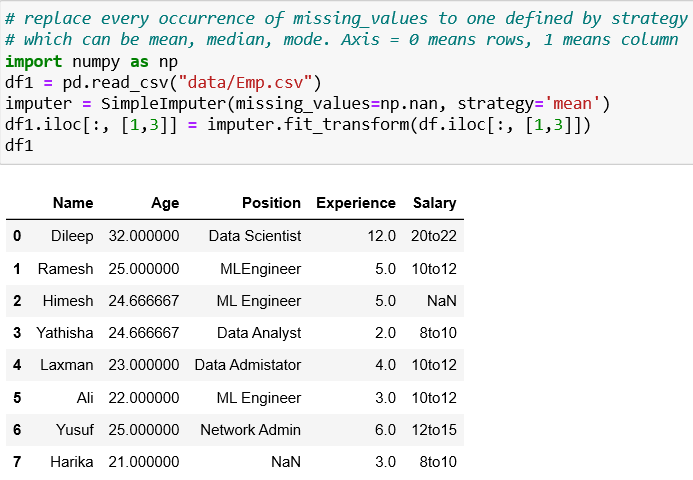2. Imputing with median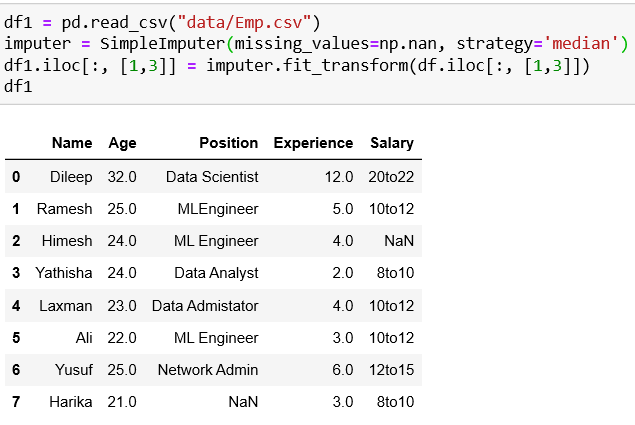3. Imputing with mode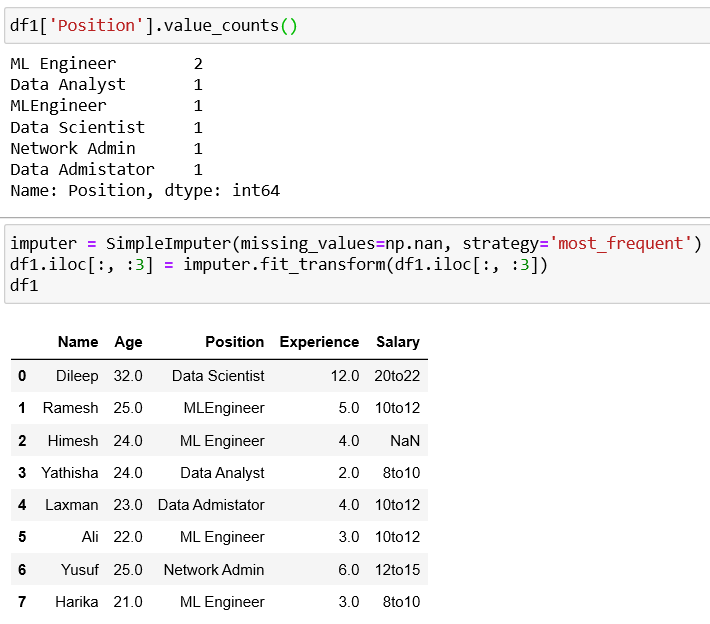4. Imputing with constant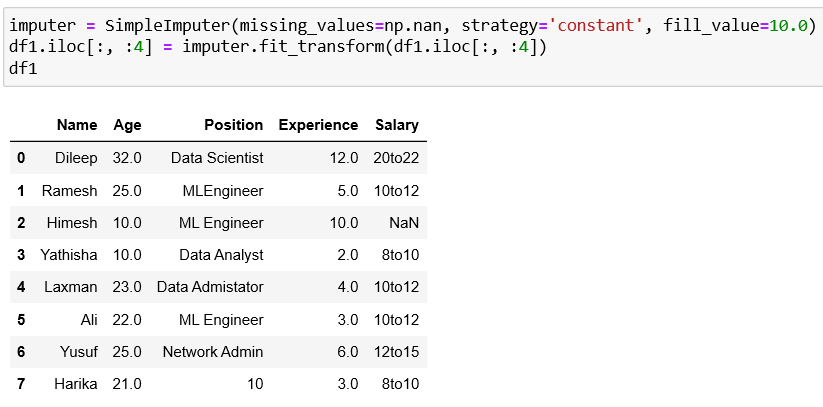Conclusion

Missing data arise in almost all serious data analyses. The approach to deal with missing values is heavily dependent on the nature of such data. Thanks for reading.